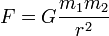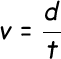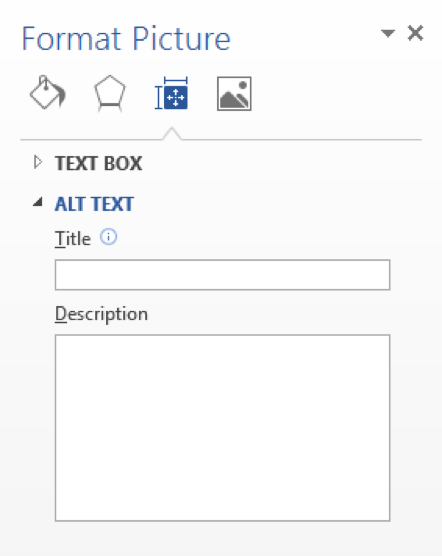# Creating Accessible Equations in MS Word

### Equations in HTML documents

In HTML, there are two ways to display equations:

1. Use ASCII character-encoding to display the symbols in the equation. For example, ∑ is displayed by the characters `& #8721;`
2. Use an image of the equation

### Equations in Word documents

When including equations in your word documents you must do all of the following:

• Insert the image (you can use a screenshot) or the equation into your word document. Send the image, if applicable, as a separate file (jpeg, jpg, gif, png, etc.)
• Provide a text description (Alt text) for the equation.

### Why Use Alt Text For Equations?

Each equation must have a text description called alternate text (Alt text) to comply with federal requirements. Section 508 of the Rehabilitation Act requires that all electronic and information technology for federal agencies is accessible to people with disabilities.

Alt text gives semantic meaning to the equation, provides a description of the image, and also provides Screen Readers with information to inform vision-impaired students of what equation they would be viewing.

Examples of Alt Text for Equations:

1. This is the original equation as an image:Alt text options:
• The force of gravity is proportional to the product of the two masses and inversely proportional to the square of the distance between them.
• F equals G times m sub 1 times m sub 2 over r squared, where F is the force between the masses, G is the gravitational constant, m1 is the first mass, m2 is the second mass, and r is the distance between the masses.
• F equals G times m sub 1 times m sub 2 over r squared.
2. This is the original equation as an image:Alt text options:
• Velocity is equal to distance divided by time.
• V equals d over t, where v is velocity, d is distance, and t is time.
• V equals d over t.

Note: Alt text is not currently available in Word 2008 or 2011. Please provide a caption above the image (e.g., Image below states that V equals d over t, where v is velocity, d is distance, and t is time).

### To add an equation image in Word (Windows)

1. Click on the Insert tab.
2. Select Picture.
3. Locate and select the image on your computer.
4. Click the Insert button.
5. Right click on the image.
6. Select Format Picture.
7. Select Format Picture
8. Click the cross icon for Layout & Properties
9. Click the drop-down for ALT TEXT10. Add a title and description of the image in the Alternative text box.

### To add an equation image in Word (Mac)

1. Click on Home on the menu bar.
2. Select Picture, then Picture from File…
3. Locate and select the image on your computer.
4. Click the Insert button.
5. Since alt text is not available in Word 2011, provide a caption below the image (e.g., Image below of Instructor Mr. Smith).